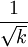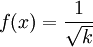# A Second Motivating Problem for The Integral Test

Previous: A Motivating Problem for The Integral Test

Next: Theorem: The Integral Test

## Example

Now let's try to determine whether the following seriesconverges. We can use the same approach that we took withto obtain our result, which as you might guess, will show that this series is divergent.

## Relating Our Series to an Integral

To introduce an integral, we must introduce a function to work with. Using the same approach with our previous example, because the general term of our series isso let's try the functionNote that, once again this function meets the following criteria:

• f(x) is greater than zero for all x
• f(x) is continuous
• f(x) is a decreasing functionStarting with the equilateral triangle, this diagram gives the first three iterations of the Koch Snowflake (Creative Commons, Wikimedia Commons, 2007).

As in the previous example, we look to a geometrical interpretation of what we are doing. The Figure 1 gives us a graph of f(x) = 1/x2 along with a set of rectangles. Notice that the areas of the rectangles are greater than area underbecause of the way we constructed the rectangles.

However, it is a known result, often covered in an integral calculus course, that the integralis divergent. Because the rectangle area is greater than the area of the curve, the rectangle area must be infinite as well. So the given sequence must be divergent.

Previous: A Motivating Problem for The Integral Test

Next: Theorem: The Integral Test